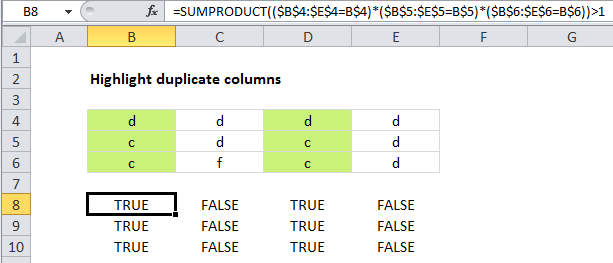# Highlight duplicate columns in Excel

This tutorial shows how to  Highlight duplicate columns in Excel using the example below;

## Formula

`=SUMPRODUCT((row1=ref1)*(row2=ref2)*(row3=ref3))>1`## Explanation

Excel contains a built-in preset for highlighting duplicate values with conditional formatting, but it only works at the cell level. If you want to find and highlight duplicate columns, you’ll need to use your own formula, as explained below.

Also See:   Excel Data validation must not contain

To highlight duplicate columns, you can use a formula based on the SUMPRODUCT function.  In the example shown, the formula used to highlight duplicate columns is:

`=SUMPRODUCT((\$B\$4:\$E\$4=B\$4)*(\$B\$5:\$E\$5=B\$5)*(\$B\$6:\$E\$6=B\$6))>1`

### How this formula works

This approach uses SUMPRODUCT to count the occurrence of every value in the table, one row at a time. Only when the same value appears in the same location in all three rows is a count generated. For cell B4, the formula is solved like this:

```=SUMPRODUCT((\$B\$4:\$E\$4=B\$4)*(\$B\$5:\$E\$5=B\$5)*(\$B\$6:\$E\$6=B\$6))>1
=SUMPRODUCT(({1,1,1,1})*({1,0,1,0})*({1,0,1,0}))>1
=SUMPRODUCT({1,0,1,0})>1
=2>1
=TRUE```

Note that row references are fully absolute, while cell references are mixed, with only the row locked.

Also See:   Count if row meets internal criteria in Excel

### With a helper row

If you don’t mind adding a helper row to your data, you can simplify the conditional formatting formula quite a bit. In a helper row, concatenate all values in the column. Then you can use COUNTIF on that one row to count values that appear more than once, and use the result to trigger conditional formatting in the entire column.

Also See:   Sum by month ignore year in Excel